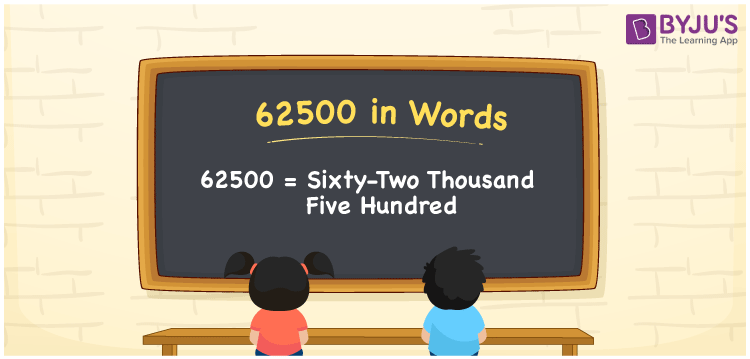# 62500 in Words

62500 in words can be written as Sixty-two Thousand Five Hundred. Here students learn about the concept of writing numbers into words using the English alphabet. For example, if you buy an AC for Rs. 62500, then you can say that “I bought an AC for Sixty-two Thousand Five Hundred Rupees”. Learn how you can write numbers in words and get a good understanding of the basic Maths concepts. So, 62500 can be read as “Sixty-two Thousand Five Hundred” in English.

 62500 in words Sixty-two Thousand Five Hundred Sixty-two Thousand Five Hundred in Numbers 62500

## 62500 in English Words## How to Write 62500 in Words?

62500 has five digits and its place value can be understood from the table given below. Using this, students will be able to answer the questions based on the place value chart of numbers confidently.

 Ten Thousands Thousands Hundreds Tens Ones 6 2 5 0 0

The expanded form of 62500 is:

6 x Ten Thousands + 2 x Thousands + 5 × Hundred + 0 × Ten + 0 × One

= 6 x 10000 + 2 x 1000 + 5 x 100

= 60000 + 2000 + 500

= 62500

= Sixty-two Thousand Five Hundred

Therefore, 62500 in words is written as Sixty-two Thousand Five Hundred.

62500 is a natural number that precedes 62501 and succeeds 62499.

62500 in words – Sixty-two Thousand Five Hundred

Is 62500 an odd number? – No

Is 62500 an even number? – Yes

Is 62500 a perfect square number? – Yes

Is 62500 a perfect cube number? – No

Is 62500 a prime number? – No

Is 62500 a composite number? – Yes

## Frequently Asked Questions on 62500 in Words

Q1

### How can 62500 be written in words?

62500 can be written in words as “Sixty-two Thousand Five Hundred”.
Q2

### Write Sixty-two Thousand Five Hundred in numbers.

Sixty-two Thousand Five Hundred can be written as 62500 in numbers.
Q3

### Is 62500 an even number?

Yes, 62500 is an even number as it is completely divisible by 2.